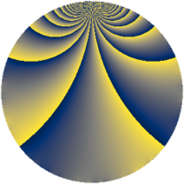# Properties

 Label 1386.2.bjLevel $1386$ Weight $2$ Character orbit 1386.bj Rep. character $\chi_{1386}(659,\cdot)$ Character field $\Q(\zeta_{6})$ Dimension $144$ Sturm bound $576$

# Related objects

## Defining parameters

 Level: $$N$$ $$=$$ $$1386 = 2 \cdot 3^{2} \cdot 7 \cdot 11$$ Weight: $$k$$ $$=$$ $$2$$ Character orbit: $$[\chi]$$ $$=$$ 1386.bj (of order $$6$$ and degree $$2$$) Character conductor: $$\operatorname{cond}(\chi)$$ $$=$$ $$99$$ Character field: $$\Q(\zeta_{6})$$ Sturm bound: $$576$$

## Dimensions

The following table gives the dimensions of various subspaces of $$M_{2}(1386, [\chi])$$.

Total New Old
Modular forms 592 144 448
Cusp forms 560 144 416
Eisenstein series 32 0 32

## Trace form

 $$144q - 8q^{3} - 72q^{4} - 12q^{5} + 24q^{9} + O(q^{10})$$ $$144q - 8q^{3} - 72q^{4} - 12q^{5} + 24q^{9} - 18q^{11} + 4q^{12} - 20q^{15} - 72q^{16} + 12q^{20} + 6q^{22} + 60q^{25} - 32q^{27} + 12q^{31} - 6q^{33} + 12q^{34} - 12q^{36} - 24q^{37} + 60q^{38} - 48q^{45} + 12q^{47} + 4q^{48} + 72q^{49} + 96q^{59} - 8q^{60} + 144q^{64} + 32q^{66} + 40q^{69} + 20q^{75} - 48q^{78} + 64q^{81} + 24q^{82} - 108q^{86} + 6q^{88} + 48q^{91} - 84q^{93} - 20q^{99} + O(q^{100})$$

## Decomposition of $$S_{2}^{\mathrm{new}}(1386, [\chi])$$ into newform subspaces

The newforms in this space have not yet been added to the LMFDB.

## Decomposition of $$S_{2}^{\mathrm{old}}(1386, [\chi])$$ into lower level spaces

$$S_{2}^{\mathrm{old}}(1386, [\chi]) \cong$$ $$S_{2}^{\mathrm{new}}(99, [\chi])$$$$^{\oplus 4}$$$$\oplus$$$$S_{2}^{\mathrm{new}}(198, [\chi])$$$$^{\oplus 2}$$$$\oplus$$$$S_{2}^{\mathrm{new}}(693, [\chi])$$$$^{\oplus 2}$$